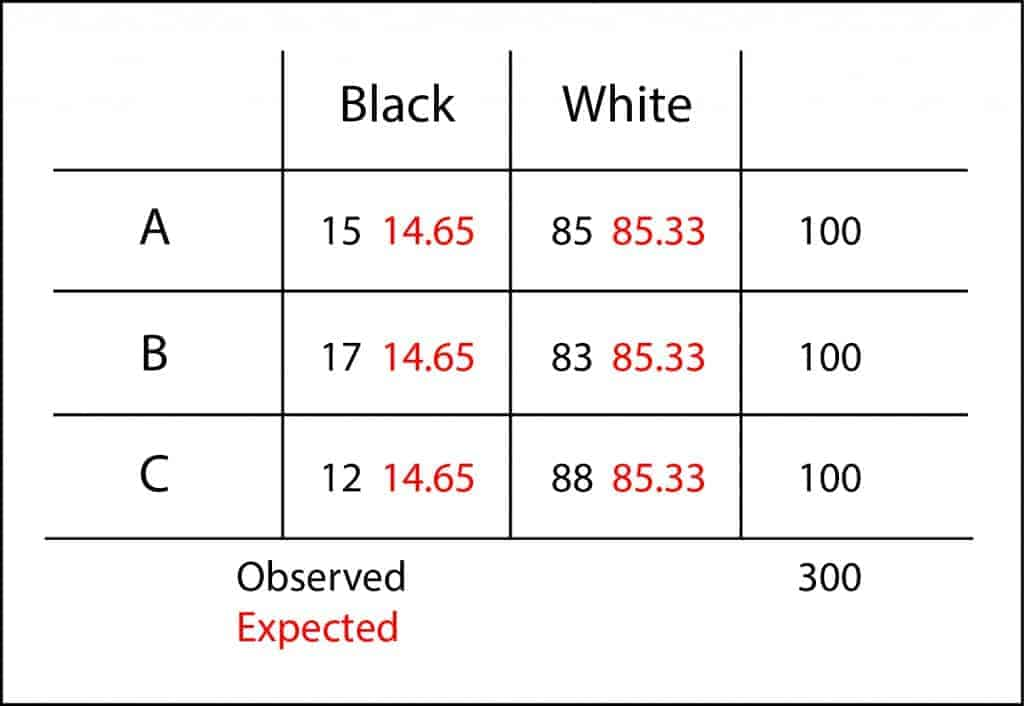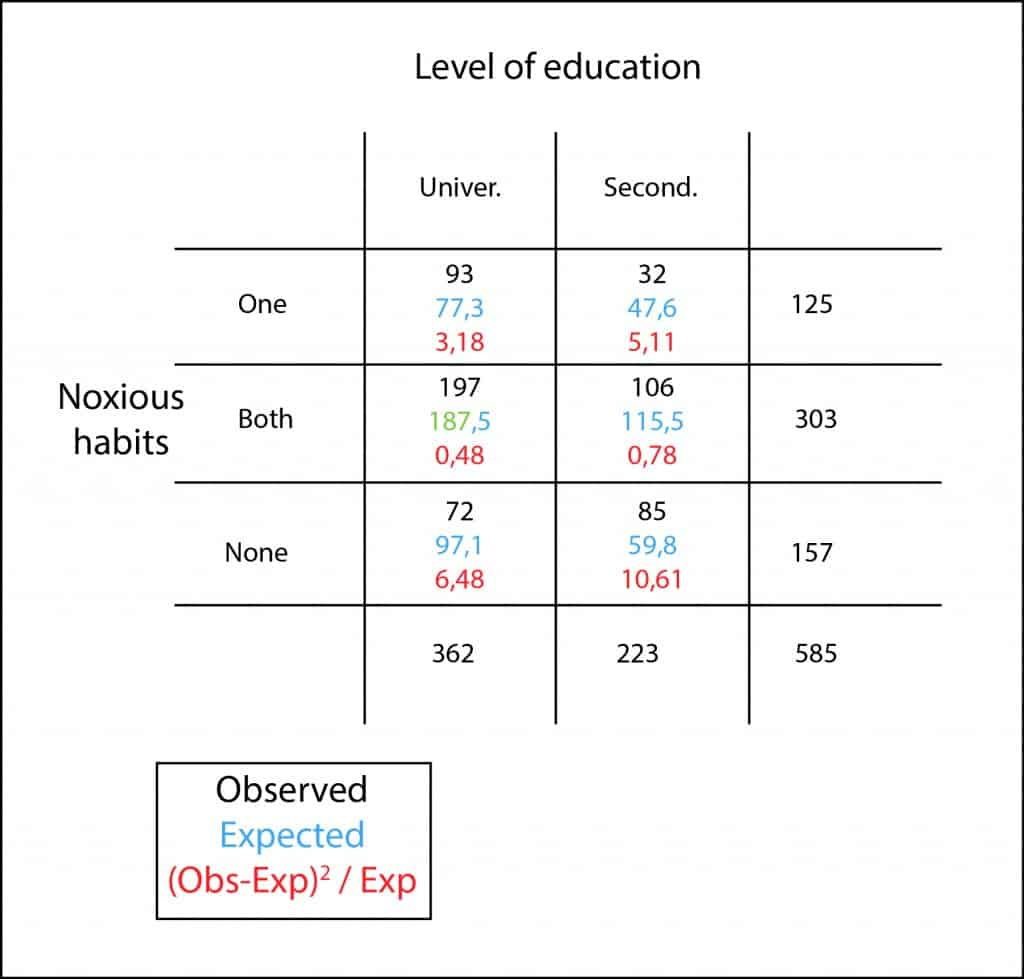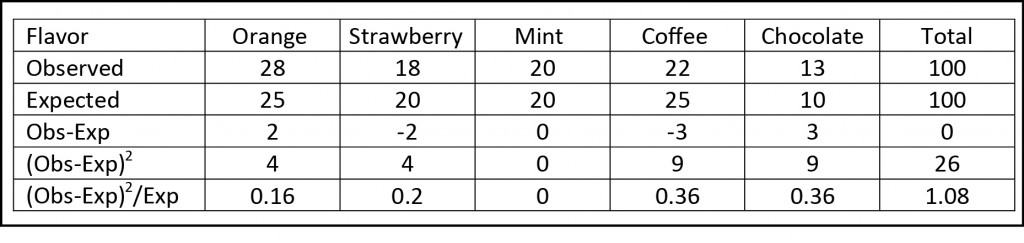## Counting sheep

There’s nothing more unfortunate that being a black sheep. We know that the term is commonly used to refer to someone who stands out in a group or a family, usually due to a negative trait. But black sheep, in the literal sense of the word, exist in the real world. And as their wool is less valued than that of white sheep, it is easy to understand the shepherd’s annoyance when he discovers a black sheep in his flock.

So, we, to compensate for some discrimination against black sheep, will count sheep, but only black. Let’s suppose that during a hallucinatory attack we decide that we want to become shepherds. We go to a cattle fair and look for a herd to buy it.

But then, as we are rookies on the business, they’ll try to sell us the herds that have more black sheep. So, we take three random samples of 100 sheep from three herds, A, B and C, and count the number of black sheep: 15, 17 and 12. Does this mean that flock C is the one with less black sheep?. We cannot be sure with these data alone. We may have, just by chance, selected a sample with less black sheep when, actually, this is the flock with more of them. As the differences are small, we may venture to think that there’re not great differences among the three herds and that the observed ones are simply due to random sampling error. This will be our null hypothesis: the three herds are similar in proportion of black sheep. We can now make our hypothesis testing.

We know that we can use the analysis of variance to compare means of different populations. This test is based on whether the differences among groups are greater than differences due to random sampling error. However, in our example, we have no means, but percentages. How can we do the hypothesis contrast?. When we want to compare counts or percentages we have to resort to the chi-square test, although the reasoning is very similar: to check if differences among expected and observed values are large enough.First, let’s build our contingency table with observed and expected values. To calculate the expected values in a cell we just have to multiply its marginal row by its marginal column and divide it by the total of the table. Whoever wants to know why this is done so, you can read the post where we explained it.

Once we have calculated observed and expected values, we calculate the differences among them. If we sum up them now, positive differences would cancel out negative ones, so we squared them before, as we do to calculate the standard deviation of a data distribution. Finally, we must standardize these differences dividing them by their expected values. It is not the same to expect one and to observe two than to expect 10 and to observe 11, although difference in both cases is equal to one. And once we have all these standardized residuals we just have to add them to obtain a value that someone dubbed as Pearson’s statistic, also known as λ.

If you do the calculation you’ll see that λ = 1.01. And that’s a lot or a little?. It so happen that λ approximately follows a chi-square probability distribution with, in our case, two degrees of freedom (rows-1 by columns-1), so we can calculate the probability to get a value of 1.01. This value is the p-value, which is 0.60. As it is greater than 0.05, we cannot reject our null hypothesis and we have to conclude that there’s not statistically significant differences among the three herds. I’d buy the cheapest of them.

These calculations can be easily done with a simple calculator, but it is usually faster to use any statistical software, especially when dealing with large contingency tables, with larger numbers or with figures with many decimal numbers.

And here we stop counting sheep. We have seen the usefulness of chi-square test to check homogeneity of populations, but chi-square is use for more things, like for testing the goodness of fit of two populations or the independence of two variables. But that’s another story…

## The why and wherefores

Do you remember the last post about girls with their level of education and their unhealthy habits?. I´ll do a brief reminder for the sake of those with a loose memory.

As it happened, we interviewed 585 girls to find out their educational level and if they smoked, drank alcohol, both of them or none. With the results obtained we built the contingency table that I attach again to show you here.We wanted to know if there was any relationship between the educational level and having bad habits, for which we began to set our null hypothesis that both qualitative variables were independent.

So we proceeded to perform a chi-square test to reach a conclusion. The first thing we have to do was to calculate the expected values of each cell, which is very ease because you just have to multiply the total of the row by the total of the column and divide the result by the total of the table… Stop right there! Why is that so?. Where does this rule come from?. Do you know why that product divided by the total equals the expected number of the cell?. It’s a good thing to have rules to facilitate our work, but I like to know where things come from and I’m sure that few of you have ever thought about it. Let’s see why.

First, let’s keep in mind that we are going to reason under the assumption of the null hypothesis that the variables harmful habits and educational level are independent. We are going to calculate the expected value of the cell corresponding to high school students with two harmful habits.

Being both situations (having studied up to secondary and both smoking and drinking) independent, the probability that both of them happen equals the product of their probabilities:

P(high school and both habits) = P(high school) x P (both habits)

We know that P(high school) will be equal to the total number of girls who studied up to high school divided by the total number of respondents to the interview. Similarly, P(both habits) will be equal to all who drink and smoke divided by the total number of respondents (total of the table). If we substitute the above expression by their values, we get:

P(high school and both habits) = (223/585) x (303/585)

We know now what is the probability of each one to belong to that cell. What is the expected number?. The answer is very simple, the probability of each one multiplied by the total number of girls interviewed:

P(high school and both habits) = 585 x (223/585) x (303/585)

If we cancel out the value 585 in numerator and denominator and simplify the expression, we’ll get:

P(high school and both habits) = (223 x 303) / 585

That is nothing more than the row’s marginal by the column’s marginal divided by the total of the table, being the result, in our example, 115.5.

So, you can see where the rule to calculate the expected number of occurrences in a contingency table comes from. Of course, you know that to find out if the variables are or not independent you still have to standardize the squared differences, calculate their sum and get the probability using a chi-square frequency distribution. But that’s another story…

## Studying or working?

I guess this phrase doesn’t have any meaning to the youngest of you or, at best, it will make you laugh as old fashioned. But I’m sure it brings back good memories to those of the same age than me, or older. The good old days when you started a conversation with this phrase, knowing you cared very little what the answer was, provided you didn’t be sent to hell. That could be the beginning of a beautiful friendship… and even more.

So as it happens that me, for better or for worse, ages have passed since the last time I said it, I’m going to invent one of my nonsense stories to have the excuse to re-use it and, incidentally, tire you out with the benefits of the chi square test. You’ll see how.

Let’s suppose that, for some reason, I want to know if the education level has any influence in having habits like smoking or drinking alcohol. So I select a random sample of 585 21-year-old women and ask them, and that’s the best: studying or working?. Thereby I classified them by education level (university and high school) and, thereafter, I check if they have one of the two habits, both or none of them. Finally, with these results I build my proverbial contingency table.We can see that, in our sample, college students have higher rates of smoking and alcohol intake. Only 19% (72 out of 362) do neither the one nor the other. This proportion rises to 38% (85 out of 223) among high school students. Therefore, consumption of tobacco and alcohol is more prevalent among the former but, can this result be extrapolated to the global population or can the observed differences be due to chance by random sampling error?. To answer this question is what we needed our chi square test for.

First, we calculate the expected values multiplying the marginal value of each cell’s row by the marginal of its column, and divide the result by the table’s total. For example, to calculate the expected value in the first cell would be (125×362) / 585 = 77.3. So, we do the same for all cells.

Once we have calculated all the expected values, what we want to know is how far away they are from the observed ones and whether this difference can be explained by chance. Of course, if we add the calculated differences, positives and negatives ones will cancel each other and the total result will be zero. This is why we use the same trick that is used to get the standard deviation: we squared the differences before adding them, disappearing negatives values as a result.

Moreover, any given difference can be more or less relevant according to its expected value. The error is greater is we expect one and get three than if we expect 25 and get 27, although difference equals two in both cases. To offset this effect we can standardize this difference dividing it by its expected value.

And now we add all these values and come up with the total sum of all cells obtaining, in our example, a value of 26.64. We just need to explain the question of whether 26.64 is too large or too small to be explained by chance.

We know that this value approximately follows a chi square frequency distribution with a number of degrees of freedom equals to (rows-1) plus (column-1), which are two in our example. So we just have to calculate the probability of that value or, what is the same, its p.

This time I’m going to do it with R, the statistical software you can find on and download from the Internet. The command for doing it is the following:

pchisq(c(26.64), df=2, lower.tail=FALSE)

We obtain a p value less than 0.001. As p < 0.05 we can reject our null hypothesis that, as usual, states that the two variables (education level and bad habits) are independent and the observed differences are due to chance.

So what does this mean?. Well, it simply means that the two variables are not independent. But no one would ever think that this result implies causality between the variables. This does not mean that studying more make you smoke or drink alcohol, but simply that the observed distribution between both variables is different from what would be expected by chance alone. This may be due to these variables or to other that we haven’t even considered. For instance, it strikes me that age in both groups might be a more logical explanation of that situation that, on the other hand, is just a product of my imagination.

And once we know the two variables are dependent, will the strength of this dependence be stronger the higher the chi or the lower the p?. Certainly not!. The higher the chi or the lower the p, the lower the probability of being wrong and commit a type 1 error. If we want to know the strength of the association we have to rely in other parameters such us relative risk or odd ratio. But that’s another story…

## Solomonic decisions

How much would not have paid King Solomon to know something more about calculating odds!. And he was quite wise. But surely, if he had had a minimal understanding of statistics, his decisions would have been much easier. And, of course, he almost certainly would not have had to cut children in half. Clear that, in this case, he would not be famous now. Historical characters are like popular festivities: the wilder, the more preferred.

And to show you what I mean I’m going to imagine, as usual, an example so stupid that you will end up wanting to keep reading.

Let’s suppose for a delusional moment that I’m a security guard at a giant candy store. Someone tell me that a child has been caught with a bag of candy that has been allegedly stolen from the giant barrel full of candy in the store. The poor kid says he has done nothing wrong and that he has bought the bag at another store but, of course, what could he say?. What could we do?. I know… split the child in half, as would King Solomon.

But anyone immediately realizes that this solution is not a good one. Who knows?, the poor child could be innocent, as he claims. So let’s think a little about how we can find out if the candies come from our shop or from ours competitors.

The store clerk tell us that in the barrel 25% of the candies are orange flavor, 20% strawberry, 20% mint, 25% coffee and 10% chocolate. So we look into the child’s bag and find out that it has 100 candies of the following flavors: 27 orange, 18 strawberry, 20 mint, 22 coffee and 13 chocolate.

If those candies were from our barrel, the flavor distribution would be the same in both the barrel and the bag. From a practical point of view, we may assume that the robber pulled out 100 random candies from the barrel (we can’t follow this reasoning if he has selected the candies by its flavors).

So the question is simple: does the bag’s distribution of flavors support the candies come from a random sample of our barrel?. Small differences would be due to sampling error, so we state our null hypothesis that the kid has stolen our candy.

First, we think about the theoretical distribution that would have to have the candy and compare it with the distribution they have, always assuming that null hypothesis is true.We want to know if the difference between expected and observed distributions can be explained by chance. But if we add the differences between them they cancel each other and the end result is zero. As we know this is always going to happen, what we do is square the differences (to eliminate negative signs) before adding them. The problem is that it is not the same to expect 2 and get 7 than to expect 35 and get 40. Although the difference equals five in both examples, it seems clear that margin of error is greater in the first case. This is why we standardize the differences dividing them by the expected value. And, finally, we add these results to obtain a certain value, which in our example is 1.08.

And, 1.08 is a lot or a few?. It depends, sometimes it will be a lot and others a few. But we do know that this value approximately follows a chi-square probability distribution with a number of degrees of freedom equal to the number of categories (flavors in our example) minus one.

Know we can calculate the probability of a chi value of 1.08 with four degrees of freedom. We can use a computer program, a table of probabilities or one of the available calculators on the Internet. We come up with a p value of 0.89 (89%). As is greater than 5%, we cannot reject the null hypothesis, so we conclude that the child is not only a thief, but also a liar. His bag of candy is representative of a random sample obtained from our barrel.

You have seen how easy it is to check the origin of a sample by applying the chi-square test. But this test is not only good for studying the origin of a random sample. It can also be used to check if there is any dependence among qualitative variables. But that’s another story…